# Calculator

Of course there is a calculator on Windows. Some keyboards even have a key that will start it up.

But sometimes you need to do math on a specific field. It would be nice if the current value of the field loaded into a calculator – and when you finished the math –  the result would load back to the starting field.

Or, maybe you would just like to start a calculator in the Telios program…

On any Telios program, there are two key sequences that will display a small calculator. it looks like this: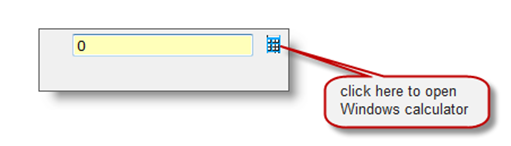#### Here is an example from the General Ledger Journal entry program: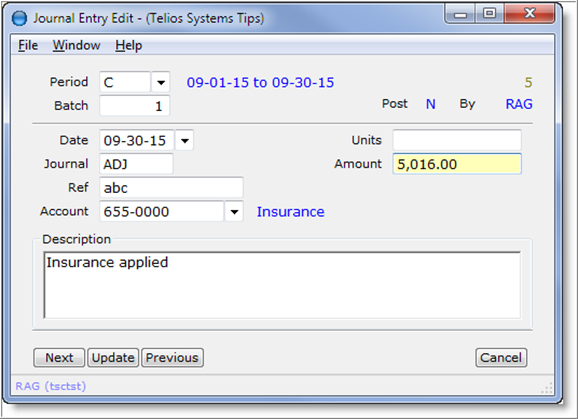The first key sequence is to press the letter C where the focus is on a numeric field – like the Amount field on this window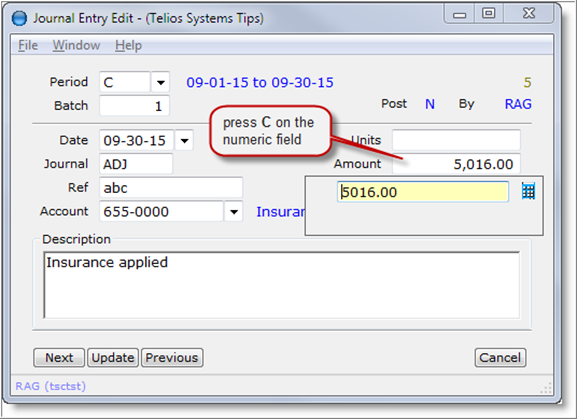The calculator will open just under the numeric field and will be loaded with the value of the field. You can press the C character anyplace on the field – beginning or end.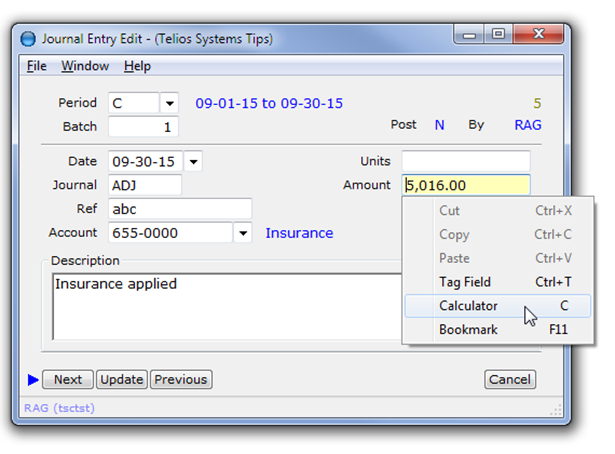You can also right-click on the numeric field to display the context menu and select Calculator C.

The second key sequence is a combination of CTRL + SHIFT + C (3 keys). This combination will work just about any place on the window. In this case, the calculator will pop up on the lower right hand corner of the window: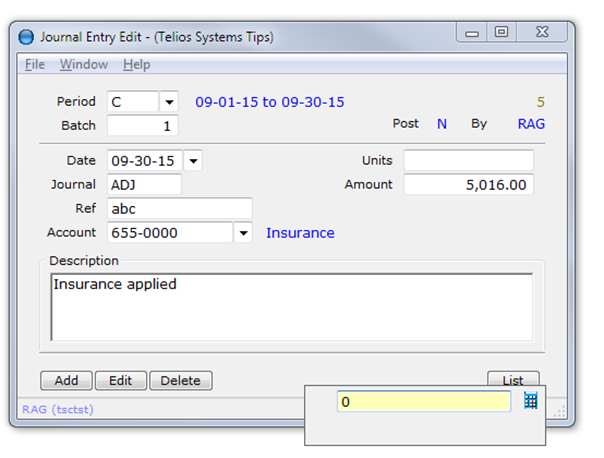With this sequence, the calculator is independent from any field in the window. However, if you press ENTER on the result of the calculation, the result will automatically copy to the clipboard, so you can paste it someplace else.

The calculator can:

• Subtract – press
• Multiply – press X or *
• Divide – press /
• Clear – press C  (or ESC if calculator field is not blank)
• Abort/Quit – press Q (quit) (or ESC if calculator field is blank)

If the calculator starts from a numeric field with C  then:

• Re-load from field – press SPACE (when calculator field is blank)
• Load result into field – press ENTER

Back to our original window, we have an insurance expense of \$5,016.00 which happens to be an annual amount. We want to divide this up to the expense for one month: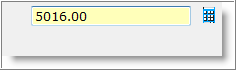press / then type 12 and ENTER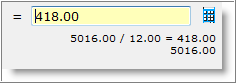For each step of the math, the operation will display below the entry.

To load the result back into the Amount field, press ENTER again.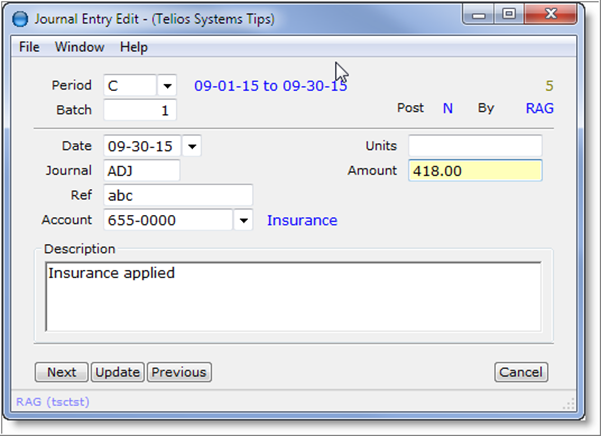The calculated amount will replace the original amount.